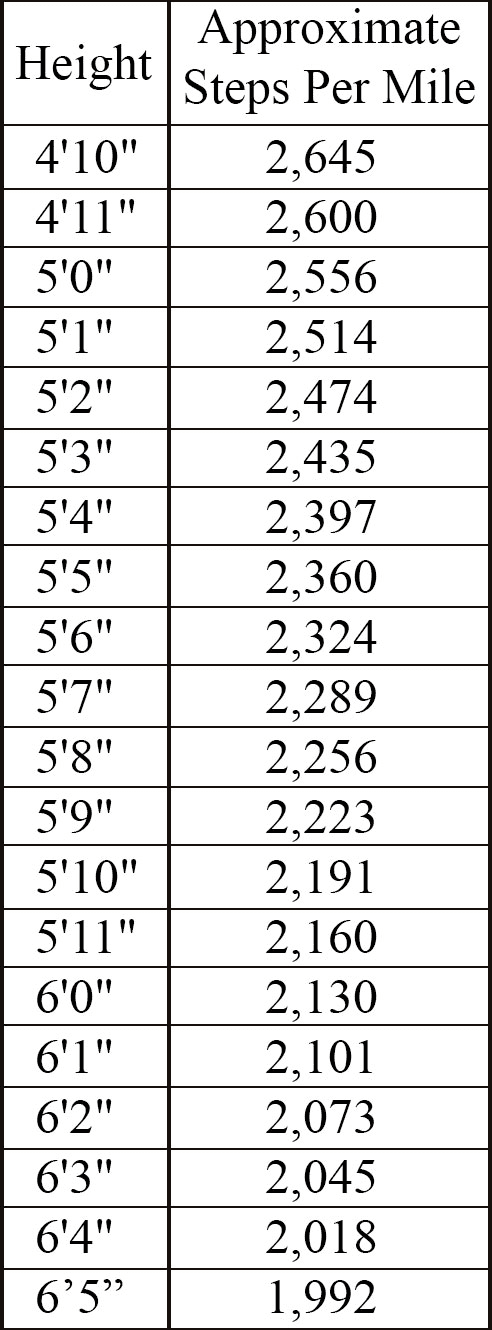# Steps Per Mile Calculator

There are a few factors to determine how many steps in a mile and these include how fast you’re going and how tall you are. Generally, the taller you are and the faster you go, the longer your stride length. If you want to figure out how many steps you walk in a mile, you don’t have to count every single step. Instead, you can base it on a shorter distance, or you can plug your height into a mathematical formula to convert steps to miles.

Here’s how to do it both ways, but if you want to skip the math altogether, jump down to the chart at the bottom to find the approximate number of steps to miles by height.

## Option 1: Count the Mile Out

Measure how many feet it takes you to walk 10 steps. Walk at a normal pace to get the most accurate number for how you usually walk.

### 2. Find Your Average Stride Length

Divide the distance it took you to walk those steps by 10. (Distance/10 = Average Stride Length in Feet.)

### 3. Find How Many Steps You Walk in a Mile

Since there are 5,280 feet per mile, divide 5,280 by your average stride length in feet. (5,280/Average Stride Length in Feet = Your Number of Steps Per Mile.)

## Option 2: Use the Mile Formula

This method may not be as accurate as measuring your exact steps. I’ll use my height as an example.

### 1. Convert Your Height to Inches

There are 12 inches in a foot. I’m 5’3”, so that’s 63 inches tall. (5 feet x 12 inches + 3 inches = 63 inches.)

### 2. Find Your Average Stride Length

Multiply your height in inches by 0.413. This is a predetermined number that figures out average stride length. Then convert your stride length back to feet for the next step. (63 inches x 0.413 = 26.019 inches per stride. 26.019 inches/12 inches per foot = 2.16825 feet per stride.)

### 3. Find How Many Steps You Walk in a Mile

Divide 5,280 by your average stride length in feet. (5,280/2.16825 feet = 2,435 steps per mile.)

Remember, stride length changes based on speed, but both of these ways provide approximate calculations to help you figure out the number of steps you take in a mile. But no matter how you calculate it, there’s one more thing to do after you figure out how many steps you take in a mile…

### 4. Get Walking!

Walking is a great way to keep your body moving, whether you do it between classes, around the office, or by running errands. Set a goal to walk a certain number of steps each day. You might be surprised at how easily they add up!Source: https://www.beachbodyondemand.com/blog/how-many-steps-walk-per-mile